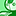# QlikView App Dev

Discussion Board for collaboration related to QlikView App Development.

Announcements
Join this live chat April 6, 10AM EST - QlikView to Qlik Sense REGISTER
cancel
Showing results for
Did you mean:Partner

## Calculated dimension in aggregated measure

Hi All,

I want to add calculated dimension in my aggregated measure.

Suppose i have calculated dimension as

=if(left(Segment,2)='KT','Apparel Knitting' ,

if(left(Segment,2)='WV','Apparel Weaving' ,

if(left(Segment,2)='HT','Home Textiles' ,

if(left(Segment,2)='TT','Technical Textile' ,

'Others'))))

now i want to use this dimension in

=sum(aggr(fieldname, 'calculated dimesion'))

5 RepliesMVP

I would recommend to do this if statement in the script so that you can use the field name within the Aggr() function....Contributor

Hi Sunitha,

If the Calculation can be added in the script instead of UI, a simple aggregation step will help to solve the issue..Partner
Author

I want to do it in front end only.

Actually i want to use this calculated dimension in my ad hoc report. I have multiple dimensions in my report (around 20 including 2 calculated dimension). i want to use all the dimensions in Aggr().Specialist II

Hi,

maybe two remarks :

- why don't use left(segment,2) as dimension (more simple as expression)  and make a pick for label ?

- why do you need aggr in chart if you have this as dimension ?

regardsMVP

I don't think there is a solution...